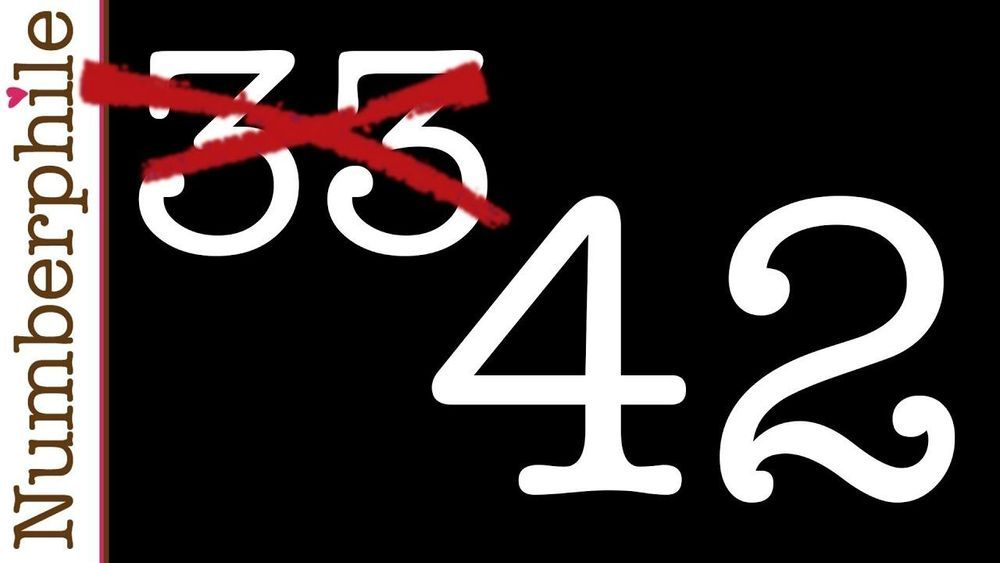## BlogA mathematician from the University of Bristol has found a solution to part of a 64-year old mathematical problem – expressing the number 33 as the sum of three cubes.

Since the 1950s, mathematicians have wondered if all could be expressed as the sum of three cubes; whether the equation k = x³+ y³+ z³ always has a .

The puzzle is a Diophantine equation in the field of , and forms part of one of the most mysterious and wickedly hard in . We still don’t know the answer.